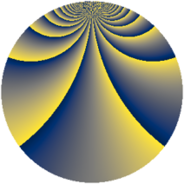# Properties

 Label 3630.2.bdLevel $3630$ Weight $2$ Character orbit 3630.bd Rep. character $\chi_{3630}(131,\cdot)$ Character field $\Q(\zeta_{22})$ Dimension $1760$ Sturm bound $1584$

# Related objects

## Defining parameters

 Level: $$N$$ $$=$$ $$3630 = 2 \cdot 3 \cdot 5 \cdot 11^{2}$$ Weight: $$k$$ $$=$$ $$2$$ Character orbit: $$[\chi]$$ $$=$$ 3630.bd (of order $$22$$ and degree $$10$$) Character conductor: $$\operatorname{cond}(\chi)$$ $$=$$ $$363$$ Character field: $$\Q(\zeta_{22})$$ Sturm bound: $$1584$$

## Dimensions

The following table gives the dimensions of various subspaces of $$M_{2}(3630, [\chi])$$.

Total New Old
Modular forms 8000 1760 6240
Cusp forms 7840 1760 6080
Eisenstein series 160 0 160

## Trace form

 $$1760q - 4q^{3} - 176q^{4} - 4q^{9} + O(q^{10})$$ $$1760q - 4q^{3} - 176q^{4} - 4q^{9} + 18q^{12} + 36q^{15} - 176q^{16} - 12q^{22} + 176q^{25} + 44q^{27} - 80q^{31} - 16q^{33} - 16q^{34} - 4q^{36} + 16q^{37} + 16q^{42} - 4q^{48} + 248q^{49} - 22q^{51} + 12q^{55} - 22q^{57} - 72q^{58} - 8q^{60} - 176q^{64} - 2q^{66} + 24q^{67} - 16q^{69} - 88q^{73} + 4q^{75} + 132q^{76} + 32q^{78} + 88q^{79} + 12q^{81} - 48q^{82} - 264q^{85} + 120q^{88} + 88q^{90} - 200q^{91} + 132q^{93} + 40q^{97} + 16q^{99} + O(q^{100})$$

## Decomposition of $$S_{2}^{\mathrm{new}}(3630, [\chi])$$ into newform subspaces

The newforms in this space have not yet been added to the LMFDB.

## Decomposition of $$S_{2}^{\mathrm{old}}(3630, [\chi])$$ into lower level spaces

$$S_{2}^{\mathrm{old}}(3630, [\chi]) \cong$$ $$S_{2}^{\mathrm{new}}(363, [\chi])$$$$^{\oplus 4}$$$$\oplus$$$$S_{2}^{\mathrm{new}}(726, [\chi])$$$$^{\oplus 2}$$$$\oplus$$$$S_{2}^{\mathrm{new}}(1815, [\chi])$$$$^{\oplus 2}$$